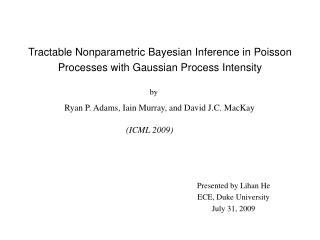Download PresentationTractable Nonparametric Bayesian Inference in Poisson Processes with Gaussian Process Intensity# Tractable Nonparametric Bayesian Inference in Poisson Processes with Gaussian Process Intensity - PowerPoint PPT Presentation

Download Presentation##### Tractable Nonparametric Bayesian Inference in Poisson Processes with Gaussian Process Intensity

Download Policy: Content on the Website is provided to you AS IS for your information and personal use and may not be sold / licensed / shared on other websites without getting consent from its author. While downloading, if for some reason you are not able to download a presentation, the publisher may have deleted the file from their server.

- - - - - - - - - - - - - - - - - - - - - - - - - - - E N D - - - - - - - - - - - - - - - - - - - - - - - - - - -
##### Presentation Transcript

1. Tractable Nonparametric Bayesian Inference in Poisson Processes with Gaussian Process Intensity by Ryan P. Adams, Iain Murray, and David J.C. MacKay (ICML 2009) Presented by Lihan He ECE, Duke University July 31, 2009

2. Outline • Introduction • The model • Poisson distribution • Poisson process • Gaussian process • Gaussian Cox process • Generating data from Gaussian Cox process • Inference by MCMC • Experimental results • Conclusion 2/19

3. Introduction Inhomogeneous Poisson process • A counting process • Rate of arrivals varies in time or space • Intensity function (s) • Astronomy, forestry, birth model, etc. 3/19

4. Introduction How to model the intensity function (s) • Using Gaussian process • Nonparametrical approach • Called Gaussian Cox process Difficulty: intractable in inference • Double-stochastic process • Some approximation methods in previous research • This paper: tractable inference • Introducing latent variables • MCMC inference – Metropolis-Hastings method • No approximation 4/19

5. Model: Poisson distribution Discrete random variable X has p.m.f. for k = 0, 1, 2, … • Number of event arrivals • Parameter  • E[X] =  • Conjugate prior: Gamma distribution 5/19

6. Model: Poisson process The Poisson process is parameterized by an intensity function such that the random number of event within a subregion is Poisson distributed with parameter for k = 0, 1, 2, … • N(0)=0 • The number of events in disjoint subregions are independent • No events happen simultaneously • Likelihood function 6/19

7. Model: Poisson process Two-dimensional spatial Poisson process One-dimensional temporal Poisson process 7/19

8. Model: Gaussian Cox process Using Gaussian process prior for intensity function (s) *: upper bound on (s) σ : logistic function g(s): random scalar function, drawn from a Gaussian process prior 8/19

9. Model: Gaussian process Definition: Let g=(g(x1), g(x2), …, g(xN)) be an N-dimensional vector of function values evaluated at N points x1:N. P(g) is a Gaussian process if for any finite subset {x1, …, xN} the marginal distribution over that finite subset g has a multivariate Gaussian distribution. • Nonparametric prior (without parameterizing g, as g=wTx) • Infinite dimension prior (dimension N is flexible), but only need to work with finite dimensional problem • Fully specified by the mean function and the covariance function Mean function is usually defined to be zero Example covariance function 9/19

10. Model: Generating data from Gaussian Cox process Objective: generate a set of event {sk}k=1:K on some subregion T which are drawn from Poisson process with intensity function 10/19

11. Inference Given a set of K event {sk}k=1:Kon some subregion T as observed data, what is the posterior distribution over (s)? Poisson process likelihood function Posterior 11/19

12. Inference Augment the posterior distribution by introducing latent variables to make the MCMC-based inference tractable. Observed data: Introduced latent variables: Total number of thinned events M Locations of thinned events Values of the function g(s) at the thinned events Values of the function g(s) at the observed events Complete likelihood 12/19

13. Inference MCMC inference: sample Sample M and: Metropolis-Hasting method Metropolis-Hasting method: draw a new sample xt+1based on the last sample xt and a proposal distribution q(x’;xt) Sample x’ from proposal q(x’; xt) 2. Compute acceptance ratio 3. Sample r~U(0,1) 4. If r<a, accept x’ as new sample, i.e., xt+1=x’; otherwise, reject x’, let xt+1=xt. 13/19

14. Inference Sample M: Metropolis-Hasting method Proposal distribution for inserting one thinned event Proposal distribution for deleting one thinned event Acceptance ratio for inserting one thinned event Acceptance ratio for deleting one thinned event 14/19

15. m Inference Sample : Metropolis-Hasting method Acceptance ratio for sampling a thinned event Sample gM+K: Hamiltonian Monte Carlo method (Duane et al, 1987) Sample *: place Gamma prior on * Conjugate prior, the posterior can be derived analytically. 15/19

16. Experimental results Synthetic data 53 events 29 events 235 events 16/19

17. Experimental results Coal mining disaster data 191 coal mine explosions in British from year 1875 to 1962 17/19

18. Experimental results Redwoods data 195 redwood locations 18/19

19. Conclusion • Proposed a novel method of inference for the Gaussian Cox process that avoids the intractability of such model; • Using a generative prior that allows exact Poisson data to be generated from a random intensity function drawn from a transformed Gaussian process; • Using MCMC method to infer the posterior distribution of the intensity function; • Compared to other method, having better result; • Having significant computational demands: infeasible for data sets that have more than several thousand event. 19/19